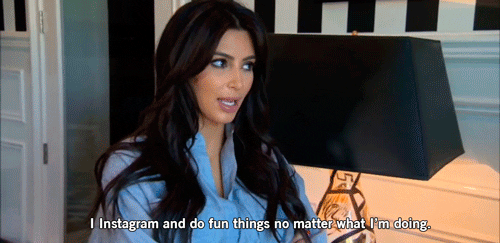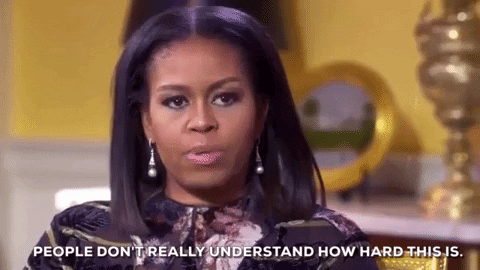•• Merch
• Donate
• SAT Prep

IntroductionIntroduction# Percentage Change

INTRO

Some of us have dreams of becoming social media influencers......and percentage change can help us understand why continuing to grow the number of followers is a lot harder than it seems.

Percentage change tells us how much the value of something changes relative to its initial value.

Imagine there are two different Instagram accounts: @almostfunlearning has followers, and @brain has followers. Overnight they both gain followers.1010,000
Technically, both users have experienced the same total change in followers (); however, @almostfunlearning has a significantly larger relative change to the start (percentage change) in followers!
To calculate percentage change we divide the change by the initial value, and multiply it by .
@almostfunlearning@brain

Check out our
Calculator
or explore our
Lesson
and
Practice

CALCULATOR

Initial
Final
KEY STEPS

## How to Calculate Percentage Change

### Step 1. Identify the initial value and the final value.

The initial value is the starting value and the final value is the ending value. It's important that we identify these correctly.

### Step 2. Calculate the change.

The change is the final value minus the initial value.

### Step 3. Calculate the change relative to the initial value.

Divide the change by the initial value. This tells us how significant of a change it is.

### Step 4. Convert the relative change to a percentage.

Multiply by by moving the decimal point two places to the right, and then add a percent sign.
Putting it all together, the full equation for percentage change is:
LESSON
Calculating Percentage Change

## Calculating Percentage Change

To calculate the percentage change, we use the following formula:

If the final value is the initial value, the percentage change will be positive.

Try it out yourself!

✨ Drag ✨

### Bigger10001000

Now, notice that when the change, or difference between the final value and the initial value, stays the same, the percentage change decreases as the initial value increases.

✨ Drag ✨

### Bigger1020

This helps us understand why it takes so much work for an aspiring influencer to maintain a continuous growth in their social media following.Kudos to the ones who make it. Shout out to the ones who try 😉👌.

### How do we solve for the final value and initial value of a percentage change?

Technically, we can use the same equation to solve for the final and initial values, but we find that using the following equation makes it a lot easier to do:

Let’s check out some step-by-step examples of calculating percentage change!
PRACTICE
Calculating Percentage Change

## Practice: Calculating Percent Change

Question 1 of 10: What is the percent change from to ?

### Step 1. Identify the initial value and the final value.

The initial value is the starting value and the final value is the ending value. It's important that we identify these correctly.

Initial:Final:

CONCLUSION
Incredible job, look at you go! Thanks for checking out this lesson ☺️🙏. Where to next?Leave Feedback